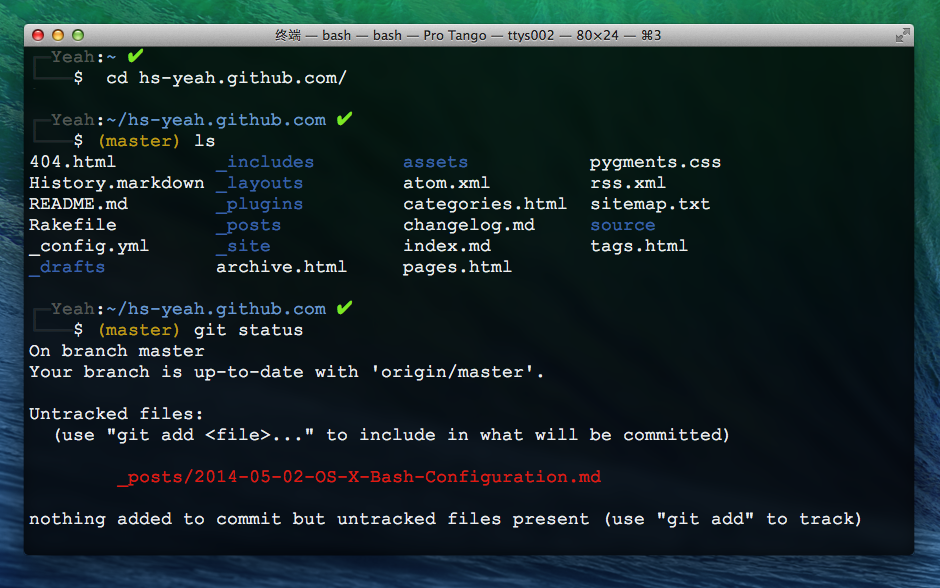02 May 2014``````export PS1='\n\[\e[0;30m\]┌─\[\e[0m\]\[\e[01;30m\]\u@\h\[\e[00m\]:\[\e[01;34m\]\w\[\e[00m\]\[\e[01;30m\] `if [ \$? = 0 ]; then echo "\[\e[32m\]✔"; else echo "\[\e[31m\]✘"; fi`\n\[\e[0;30m\]└───\[\e[0m\]\\$ \[\e[00;33m\]`git branch 2> /dev/null | grep -e ^* | sed -E  s/^\\\\\*\ \(.+\)\$/\(\\\\\1\)\/`\[\e[00m\] '
``````